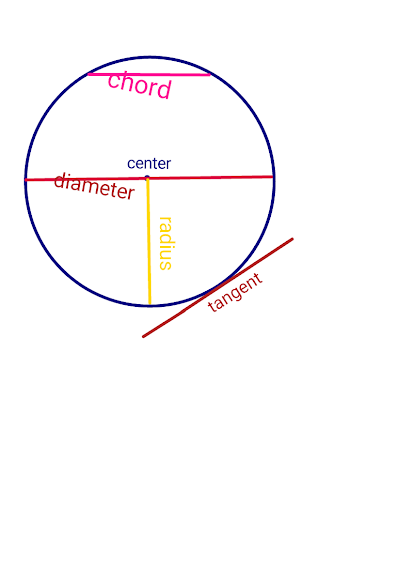# Circle Geometry

Circle GeometryCircle Geometry

## What is circle?

The locus of points (plane two dimensional) located at the same distance from a given point is called a circle. This fixed point is called the center of the circle, the distance between the center and any point of the circumference of the circle is called the radius of the circle. The circle is a simple closed curve that divides the plane into two areas: an interior and an exterior.

A circle is a type of cone (eccentricity) whose eccentricity is zero, that is, the directrix is ​​located at an infinite plane. A circle can also be defined as a special type of ellipse in which both the nuclei (Focii) coincide and the ellipse is 0. According to Euclid, ‘a circle is an unicameral plane surrounded by a line and all the lines drawn from a fixed point to that bond are equal. This bond is called the circumference and this fixed point is the center of the circle.
According to Euclid A circle is a planar shape surrounded by a bond, and it is such that all the lines up to this point at a given point are equal in length. This bond, the circumference of this circle and the fixed point, is called its center.
There is one and only 1 circle passing through 3 non-collinear points.

## What are the different parts of the circle?

Arc: Any part of the circumference of a circle.
Center: The point equidistant from all the points on the circle.
Chord: A line segment that is formed when any two points on a circle meet.
Circumference: The curve length around the circle.
Diameter: A line segment whose endpoints lie on the circle and which pass through the center or the maximum distance between any two points of the circle. It is the largest chord of the circle and it is twice the radius.
Disc: The endoplasmic region bounded by a circle.
Radius: A line segment from the center of the circle to any point in the circumference of the circle, which is half of the diameter.

Arc, Sector and Segment
Sector: An area surrounded by an arc between any two radii.
Segment: A region without center, surrounded by a chord of the circle and an arc.
Secant: An extended chord, which is the plane of the circle and intersects the circle at two points.
Tangent: The plane’s straight line that touches a circle at a point.
Semicircle: The area between the diameter of the circle and the arc between the diameter endpoints is a semicircle. The area of ​​a semicircle is half of the entire area of ​​the circle.

## What are the properties of the tangent line of the circle.

(a) At one point of the circle, there is one and only one tangent line.
(b) The tangent line of a circle is the characteristic position of the piercing line when the two ends of the corresponding chord are coincident.
(c) The common point (common point) of the tangent line and circle is called the point of contact. And the tangent line is called tangent to the common point of the circle.
(d) There is no tangent to a circle going from a point inside the circle.
(e) There is one and only one tangent to the circle from a point on the circle.
(f) There are two and only two tangent lines on a circle going from a point outside the circle.
(g) The length of the tangent line from the outer point P to the tangent point of the circle is called the length of the tangent line from the point P to the circle.

### Circumference of a circle

The circumference of the circle and its diameter are proportional to π (pi), whose value is approximately equal to 3.141592654. Thus the length of the circumference is related to C, radius r and diameter d as follows:
C=2πr=πd

### properties

• The angle subtended at any point of circumference by the diameter of the circle is a right angle (90 degrees).
• The perpendicular to the chord is also the bisector of the chord.
• The sum of the angles opposite the cyclic quadrilateral is 180 degrees.
• Two tangents can be drawn  on a circle from an external point. Both these tangents are of equal length.

### The properties of the chord of the circle

• The chord passing through the center is the largest chord of that circle. It is called ‘Diameter’.
• The diameter of a circle divides that circle into two equal parts.